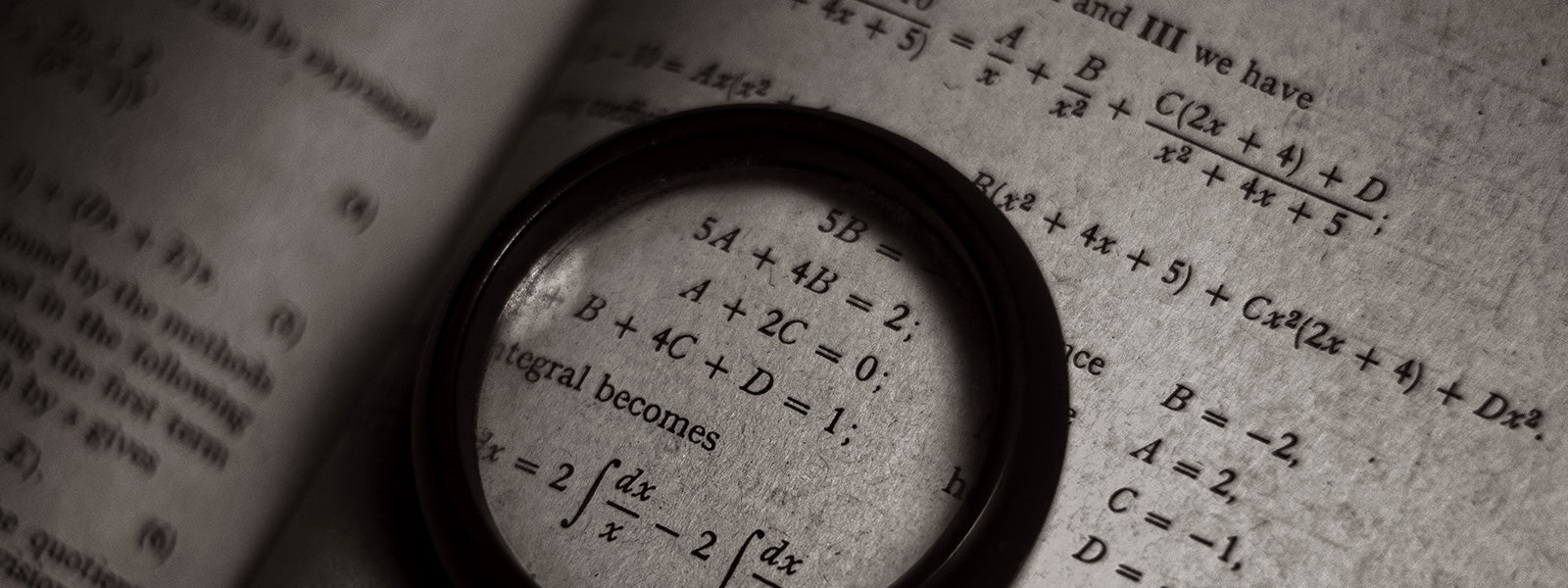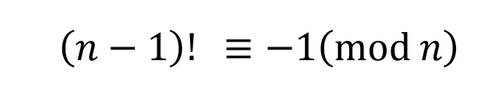We are passionate about sharing the beauty of equations in mathematics and physics and love more than anything to see customers proudly wearing (or drinking from) their Beautiful Equations apparel. However whilst we enjoy the aesthetic of such formula, much of the beauty is found in the mathematics behind the symbols. This understanding is something we would also like to share with our customers and so here is a guide to 3 of our most popular equations!

Euler’s IdentityThis is arguably the most beautiful equation in mathematics, one equality that combines 5 key concepts of mathematics;

ⅇ, Euler Constant - An irrational number that is the base of the natural logarithm, ⅇ, which arises naturally in the study of calculus.

ⅈ, Defined as the square root of –1 that is used to extend the real numbers to the complex (or imaginary) numbers.

𝜋, The ratio between the circumference of a circle and its diameter.

0 and 1, They are so commonly used so may not seem special but both play significant roles in our number systems and have unique properties.

Leonard Euler was a swiss mathematician in the 18th century, his work formed foundations of modern mathematics with contributions to geometry, calculus and number theory among others and introducing much of the notation that is so widely used today. He famously proved ‘Newton’s Identities’, Fermat’s Little theorem as well as his own ‘Euler’s theorem’.

Simple Harmonic MotionThe time period of a closed mass-spring system oscillating in simple harmonic motion can be calculated using just the mass 𝑚 and spring constant of the spring, 𝑘.

Simple harmonic motion is a type of oscillation where the restoring force on an object is directly proportional to the displacement of the object from equilibrium. It is derived using Newtonian mechanics by considering a mass on a spring and applying Newton’s 2nd Law, 𝐹=𝑚𝑎, and Hooke’s Law, 𝐹=𝑘𝑥 where 𝐹 is the force on the mass, 𝑎 its acceleration, 𝑥 its displacement from equilibrium and 𝑚 and 𝑘 as defined above.

While the case of a mass on a spring may seem trivial simple harmonic motion is used to model a wide range of real life problems from car shock absorbers, the vibrations of an ear drum that allows us to hear to bungee jumping!

Wilsons TheoremWilsons Theorem states that for any natural number n≥2, 𝑛 is prime if and only if it divides the number one greater than the product of the integers from 1 to 𝑛−1.

The biconditional statement of ‘if and only if’ makes Wilson’s Theorem a straightforward, although quite long computationally when working with large numbers, method of determining if a number is prime. It was first stated by Ibn al-Haytham, an Arab mathematician best known for his contributions to the field of optics, in around 1000AD and then again by John Wilson, the English mathematician after whom it is named, in the mid 1700s. It was however Joseph- Louis Lagrange who finally provided the proof in 1771.

- Lucy Chats Maths